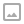## 「CF68D」Half-decay tree-期望瞎搞题

·  ✏️ About  805 words  ·  ☕ 2 mins read · 👀... views

1. add v e （ $1 \le v \le 2^{h+1}-1,1 \le e \le 10^4$ ）表示给第 $v$ 个节点的权值加 $e$ 。
2. decay 操作。我们在这个二叉树里面以等概率选择一个叶子节点，将这个叶子节点到根的路径上所有的边都删去。在删除后，树会形成若干个联通块，我们定义某个联通块的的权值为这个联通块内所有节点的权值之和。我们定义删除后的树的权值为形成的所有联通块的权值的最大值。请你求出这个值的期望。每次删除后会恢复所有删除的边。

Codeforces

## 代码

  1 2 3 4 5 6 7 8 9 10 11 12 13 14 15 16 17 18 19 20 21 22 23 24 25 26 27 28 29 30 31 32 33 34 35 36 37 38 39 40 41 42 43 44 45 46 47 48 49 50 51 52 53 54 55 56 57 58 59 60 61 62 63 64 65 66 67 68 69 70  #include using namespace std; const int MAXN = 110000,LOGN = 31; namespace Tree{ int sumn[MAXN*LOGN],ls[MAXN*LOGN],rs[MAXN*LOGN],cnt = 1; void upd(int nown,int v){ static int tmp;int ecnt = 0; for(int i = nown;i;i>>=1) tmp[++ecnt] = i&1; int now = 1; for(int i = ecnt-1;i>=0;--i){ sumn[now] += v; if(tmp[i] == 0){ if(!ls[now]) ls[now] = ++cnt; now = ls[now]; } else{ if(!rs[now]) rs[now] = ++cnt; now = rs[now]; } } } double decay(){ int now = 1,nowmax = -1e9; double ans = 0, p = 1; while(now != 0){// now 非空 int lson = sumn[ls[now]],rson = sumn[rs[now]],val = sumn[now] - lson - rson; p *= 0.5; ans += p * max(nowmax,max(lson,rson)+val); if(lson > rson){ nowmax = max(nowmax,rson+val); now = ls[now]; } else{ nowmax = max(nowmax,lson+val); now = rs[now]; } } ans += p * nowmax; return ans; } } int h,q; void init(){ scanf("%d %d",&h,&q); } void solve(){ for(int i = 1;i<=q;i++){ char s; int a,b; scanf("%s",s); if(s == 'a'){ scanf("%d %d",&a,&b); Tree::upd(a,b); } else if(s == 'd'){ printf("%.8lf\n",Tree::decay()); } } } int main(){ init(); solve(); return 0; }WRITTEN BY
cqqqwq
A student in Computer Science.